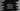# Difference between %d and %i format specifiers in C## Difference between %d and %i format specifiers in C:

Format specifiers are used to specify the format and type of data that we are dealing with. For example, format specifier %c is used to read a character value using scanf and write a character value using printf.

Similarly, we can use %d or %i to read and write numbers. But there is a difference between them. In this post, I will show you the difference between %d and %i with an example.

### Actual meanings of %d and %i:

• %d is used for signed decimal integers and %i is used for integers.
• Using %d, we can read or print positive or negative decimal values.
• Using %i, we can read or print integer values in decimal, hexadecimal or octal type.
• The hexadecimal value should starts with 0x and the octal value should starts with 0.

### Example:

Let’s take a look at the example below:

``````#include <stdio.h>

int main()
{
int firstInt = 20;
int secondInt = 25;
int octal = 020;

printf("firstInt : %d\n", firstInt);
printf("secondInt : %i\n", secondInt);
printf("octal : %i\n", octal);

return 0;
}``````

It will print:

``````firstInt : 20
secondInt : 25
octal : 16``````
• The first printf prints the decimal value of firstInt, which is 20.
• The second printf prints the decimal value of secondInt, which is 25.
• The third printf prints the decimal value of hexadecimal, which is 10, decimal representation of hexadecimal value 0xA.
• The third printf prints the decimal value of octal, which is 16, decimal representation of octal value 20.

We can also use these format specifiers with scanf to read user inputs.

``````#include <stdio.h>

int main()
{
int firstInt;
int secondInt;
int octal;

printf("Enter an integer value : ");
scanf("%d", &firstInt);

printf("Enter an integer value : ");
scanf("%i", &secondInt);

printf("Enter a hexadecimal value : ");

printf("Enter an octal value : ");
scanf("%i", &octal);

printf("firstInt : %d\n", firstInt);
printf("secondInt : %i\n", secondInt);
printf("octal : %i\n", octal);

return 0;
}``````

It will give output as like below:

``````Enter an integer value : 10
Enter an integer value : 20
Enter a hexadecimal value : 0xB
Enter an octal value : 20
firstInt : 10
secondInt : 20